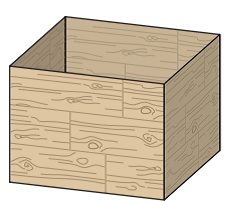Chapter 7.5, Problem 47E### Calculus: An Applied Approach (Min...

10th Edition
Ron Larson
ISBN: 9781305860919

#### Solutions

Chapter
Section### Calculus: An Applied Approach (Min...

10th Edition
Ron Larson
ISBN: 9781305860919
Textbook Problem
1 views

# Cost A manufacturer makes an open-top wooden crate having a volume of 18 cubic feet. Material costs are $0.20 per square foot for the base and$0.15 per square foot for the sides. Find the dimensions that minimize the cost of each crate. What is the minimum cost?To determine

To calculate: The dimensions that minimize the cost of each crate and the minimum cost. If The volume of an open-top wooden crate is 18 cubic feet. the cost of material is $0.20 per square foot for the base and$0.15 per square foot for the side.

Explanation

Given Information:

The provided figure is,

Formula used:

Consider a package (rectangular) of the following dimensions,

Then the volume is V=lbh.

Also, if there is a function f in a variable x, it’s critical point can be found by taking a derivative and setting it equal to zero,

fx=0

Step1: 2fx2>0 for the above critical point then f attains a minimum.

Step2: 2fx2<0 for the above critical point then f attains a maximum.

Calculation:

Let the length of the rectangular package be x, width be y and height be z.

Since, the volume of an open-top wooden crate is 18 cubic feet. so,

xyz=18

It can be written as z=18xy.

The cost of each crate is

C=0.2xy+0.15(2)xz+0.15(2)yz=0.2xy+0.3xz+0.3yz=0.2xy+0.3x(18xy)+0.3y(18xy)=0.2xy+5.4y+5.4x

Now find partial derivative of V with respect to y and z,

Cx=0.2y5

### Still sussing out bartleby?

Check out a sample textbook solution.

See a sample solution

#### The Solution to Your Study Problems

Bartleby provides explanations to thousands of textbook problems written by our experts, many with advanced degrees!

Get Started

#### 23/2

Applied Calculus for the Managerial, Life, and Social Sciences: A Brief Approach

#### In Problems 7-34, perform the indicated operations and simplify. 19.

Mathematical Applications for the Management, Life, and Social Sciences

#### Given the Taylor Series , a Taylor series for ex/2 is:

Study Guide for Stewart's Multivariable Calculus, 8th

#### Sometimes, Always, or Never: If for all n and both {an} and {cn} converge, then{bn} converges.

Study Guide for Stewart's Single Variable Calculus: Early Transcendentals, 8th